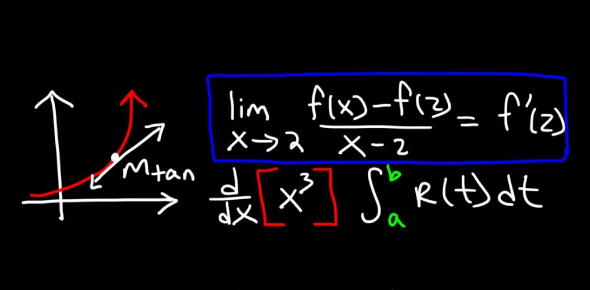# Integration Methods Quiz: Calculus Mathematics

10 Questions | Total Attempts: 89SettingsHave you studied Calculus mathematics? If yes, you can play this Integration Methods Quiz to check your knowledge of various methods of Integration. You can get the perfect score just by answering all the questions correctly. All the best for your mathematics results.

• 1.
Which statement best describes the meanings of definite and indefinite integrals?
• A.

Definite and indefinite integrals represent areas under curves. Definite integrals are for a specific interval, while indefinite integrals are accumulation functions.

• B.

Definite integrals represent backwards differentiation while indefinite integrals represent limits of Riemann sums.

• C.

Definite integrals represent areas under curves while indefinite integrals represent families of antiderivatives.

• D.

Definite integrals represent families of antiderivatives while indefinite integrals represent specific antiderivatives.

• 2.
Which two scientists were involved in the discovery of "the Fundamental Theorem of Calculus?"
• A.

Sir Isaac Newton

• B.

Albert Einstein

• C.

Gottfried Wilhelm Leibniz

• D.

Srinivasa Ramanujan

• 3.
Who introduced the notation for the indefinite integral?
• A.

Jacob Bernoulli

• B.

Joseph Fourier

• C.

Gottfried Leibniz

• D.

Georg Riemann

• 4.
Which method is used to find integration by introducing an independent variable?
• A.

Integration by Substitution

• B.

Integration Using Trigonometric Identities

• C.

Integration by Partial Fraction

• 5.
Which method involves ILATE rule?
• A.

Integration Using Trigonometric Identities

• B.

Integration by Parts

• C.

Integration by Partial Fraction

• 6.
If any integrand have trigonometric functions, then Trigonometric Identities method is used.
• A.

True

• B.

False

• 7.
Partial fraction method is used when the ration of two polynomials is there.
• A.

True

• B.

False

• 8.
There are 3 forms of partial fraction.
• A.

True

• B.

False

• 9.
The forms of partial fraction
• A.

Trigonometric

• B.

Proper

• C.

Substitution

• D.

Improper

• 10.
Integration of Some particular function: various standard integrals that can be integrated using the important formulas of integration
• A.

True

• B.

False

Related TopicsBack to top